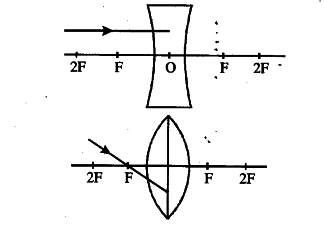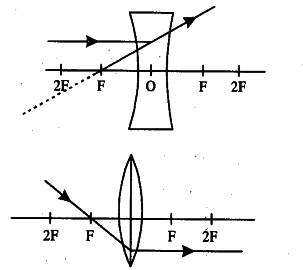# The refractive index of diamond is 2.42. What is the meaning of this statement?

(a) The refractive index of diamond is 2.42. What is the meaning of this statement?
(b) Redraw the diagram given below in your answer book and complete the path of ray.© What is the difference between virtual images produced by concave plane and convex mirrors ?
(d) What does the negative sign in the value of magnification produced by a mirror indicates about a image?

(a) This means that the ratio of speed of light in air/vacuum and speed of light in diamond is 2.42.
(b) The two figures are drawn below :© Virtual image produced by concave mirror is magnified; that produced by plane mirror is of the same size and the .virtual image produced by convex mirror is diminished.
(d) It indicates that the image is real.